# Olympiad Test: Triangle - 1

## 15 Questions MCQ Test Mathematics Olympiad Class 7 | Olympiad Test: Triangle - 1

Description
Attempt Olympiad Test: Triangle - 1 | 15 questions in 30 minutes | Mock test for Class 7 preparation | Free important questions MCQ to study Mathematics Olympiad Class 7 for Class 7 Exam | Download free PDF with solutions
QUESTION: 1

### Two angles of a triangle are equal and the third angle measures 70°. Find the measure of each of the unknown angles.

Solution:

Let the measure of each unknown angle be x°. We know that the sum of the angles of a triangle is 180°.
∴ x + x + 70 = 180°
⇒ 2x = (180° – 70°)
⇒ 2x = 110°
⇒ x = 55°
Hence each unknown angle is 55°.

QUESTION: 2

### In a ∆XYZ, if ∠X = 90° and ∠Z = 48°, find ∠Y.

Solution:

We know that the sum of the angles of a triangle is 180°.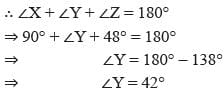QUESTION: 3

### Each of the two equal angles of an isosceles triangle is twice the third angle. Find the angles of the triangle.

Solution:

Let the third angle be x°.
We know that the sum of the angles of a triangle is 180°.
∴ 2x + 2x + x = 180°
⇒ 5x = 180°
⇒ x = 36°
So, the angles are (2 × 36)°, (2 × 36)° and (x)°
Hence, the angles of the triangles are 72°, 72°, 36°.

QUESTION: 4

What is the measure of each angle of an equilateral triangle?

Solution:

All the angles of equilateral triangle are equal.

QUESTION: 5

Find the angles of a triangle which are in the ratio 4 : 3 : 2.

Solution:

Let the measure of the given angles of the triangle be (4x)°, (3x)° and (2x)° respectively.
4x + 3x + 2x = 180°
⇒ 9x = 180°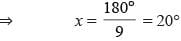So, the angles measure (4×20)°, (3 × 20)° and (2 × 20)°
Hence, the angles of the triangle are 80°, 60°, 40°.

QUESTION: 6

In the given figure, find the values of x and y.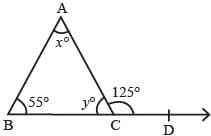Solution:

We know that an exterior angle of a triangle is equal to the sum of its interior opposite angles.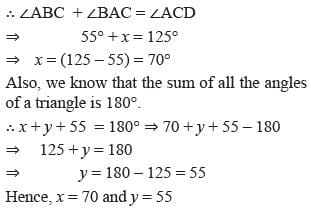QUESTION: 7

In the given figure, find the values of x and y.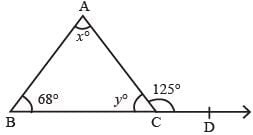Solution:

We know that an exterior angle of a triangle is equal to the sum of its interior opposite angles.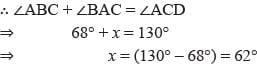The sum of all the angles of a triangle is 180°.
x + y + 68 = 180°  ⇒ 62° + y + 68° = 180°
⇒ 130° + y = 180°
y = 180° – 130°
= 50°

QUESTION: 8

In the figure given alongside, x : y = 2 : 3 and ∠ACD = 130° find the values of x, y and z.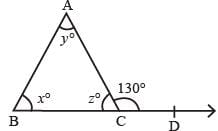Solution:

Let x = 2t, y = 3t, then 2t + 3t = 130° ⇒ t = 26°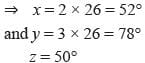∴ Sum of all angles of a triangle is 180°

QUESTION: 9

A man goes 24m due east and then 10m due north. How far is he away from his initial position?

Solution:

Let O be the initial position of the man. Let he cover OA = 24m due east and then AB = 10m due north.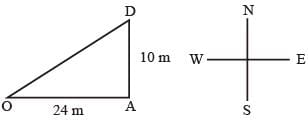Finally, he reaches the point B. Join OB.
OB2 = (OA2 + OB2) = {(24)2 + (10)2} m2
= (576 + 100) m2 = 676 m2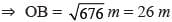Hence, the man is at a distance at 26m from his initial position.

QUESTION: 10

The lengths of the sides of two triangles are given below. Which of them is right – angled?
(i) a = 8 cm, b = 5 cm, and c = 10 cm
(ii) a = 7 cm, b = 24 cm, and c = 25 cm

Solution:

(i)
Here a  = 8 cm, b = 5cm  and c = 10c,
The largest side is c = 10cm.
a2 + b2 = {(8)2 + (5)2} cm2
= m (64 + 25) cm2 = 89 cm2 ≠ (10)2 cm2
a2 + b2 ≠ c2
∴ Given triangle is not right angled.
(ii) Here a = 7 cm, b = 24 cm and c = 25 cm
The largest side is c = 25 cm
a2 + b2 = {(7)2 + (24)2} cm2 = (49 + 576) cm2
= 625 cm2 = (25 cm)2 = c2
⇒ a2 + b2 = c2
Given triangle is right angled.

QUESTION: 11

Two poles of height 9 cm and 14m stand upright on a plane ground. If the distance between their feet is 12 m. Find the distance between their tops.

Solution:

Let AB and CD be the given poles such that
AB = 9 m, CD = 14 m and AC = 12 m. Join BD.
From B, draw BL ⊥ CD.
DL = (CD – CL) = (CD – AB)
= (14 – 9) m = 5 m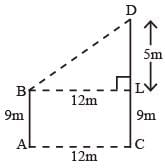BL = AC = 12 m
Now, in right ∆BLD, by Pythagoras theorem.
We have
BD2 = BL2 + DL2  = {(12)2 + (5)2}m2
= (144 + 25) m2 = 169 m2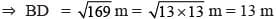QUESTION: 12

Two circles are congruent if they have

Solution:
QUESTION: 13

Two lines segments are congruent if they have

Solution:
QUESTION: 14

In a ∆ABC it is given that ∠B = 37° and ∠C = 29°. Then ∠A = ?

Solution:

We know that the sum of the angles of  a triangle is 180°.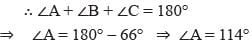QUESTION: 15

In a ∆ABC, if  2∠A = 3, ∠B = 6∠C then ∠B = ?

Solution: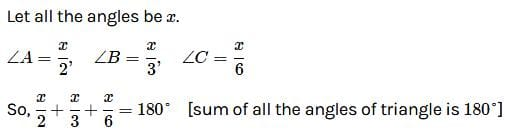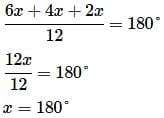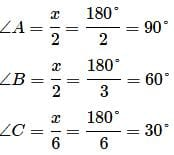Use Code STAYHOME200 and get INR 200 additional OFF Use Coupon Code